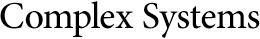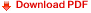## Simulated Binary Crossover for Continuous Search SpaceKalyanmoy Deb
Ram Bhushan Agrawal
Department of Mechanical Engineering,
Indian Institute of Technology,
Kanpur, UP 208 016, India,

#### Abstract

The success of binary-coded genetic algorithms (GAs) in problems having discrete search space largely depends on the coding used to represent the problem variables and on the crossover operator that propagates building blocks from parent strings to children strings. In solving optimization problems having continuous search space, binary-coded GAs discretize the search space by using a coding of the problem variables in binary strings. However, the coding of real-valued variables in finite-length strings causes a number of difficulties: inability to achieve arbitrary precision in the obtained solution, fixed mapping of problem variables, inherent Hamming cliff problem associated with binary coding, and processing of Holland's schemata in continuous search space. Although a number of real-coded GAs are developed to solve optimization problems having a continuous search space, the search powers of these crossover operators are not adequate. In this paper, the search power of a crossover operator is defined in terms of the probability of creating an arbitrary child solution from a given pair of parent solutions. Motivated by the success of binary-coded GAs in discrete search space problems, we develop a real-coded crossover (which we call the simulated binary crossover, or SBX) operator whose search power is similar to that of the single-point crossover used in binary-coded GAs. Simulation results on a number of real-valued test problems of varying difficulty and dimensionality suggest that the real-coded GAs with the SBX operator are able to perform as good or better than binary-coded GAs with the single-point crossover. SBX is found to be particularly useful in problems having multiple optimal solutions with a narrow global basin and in problems where the lower and upper bounds of the global optimum are not known a priori. Further, a simulation on a two-variable blocked function shows that the real-coded GA with SBX works as suggested by Goldberg and in most cases the performance of real-coded GA with SBX is similar to that of binary GAs with a single-point crossover. Based on these encouraging results, this paper suggests a number of extensions to the present study.